# How to subtract multiple cells in Excel

You can watch a video tutorial here.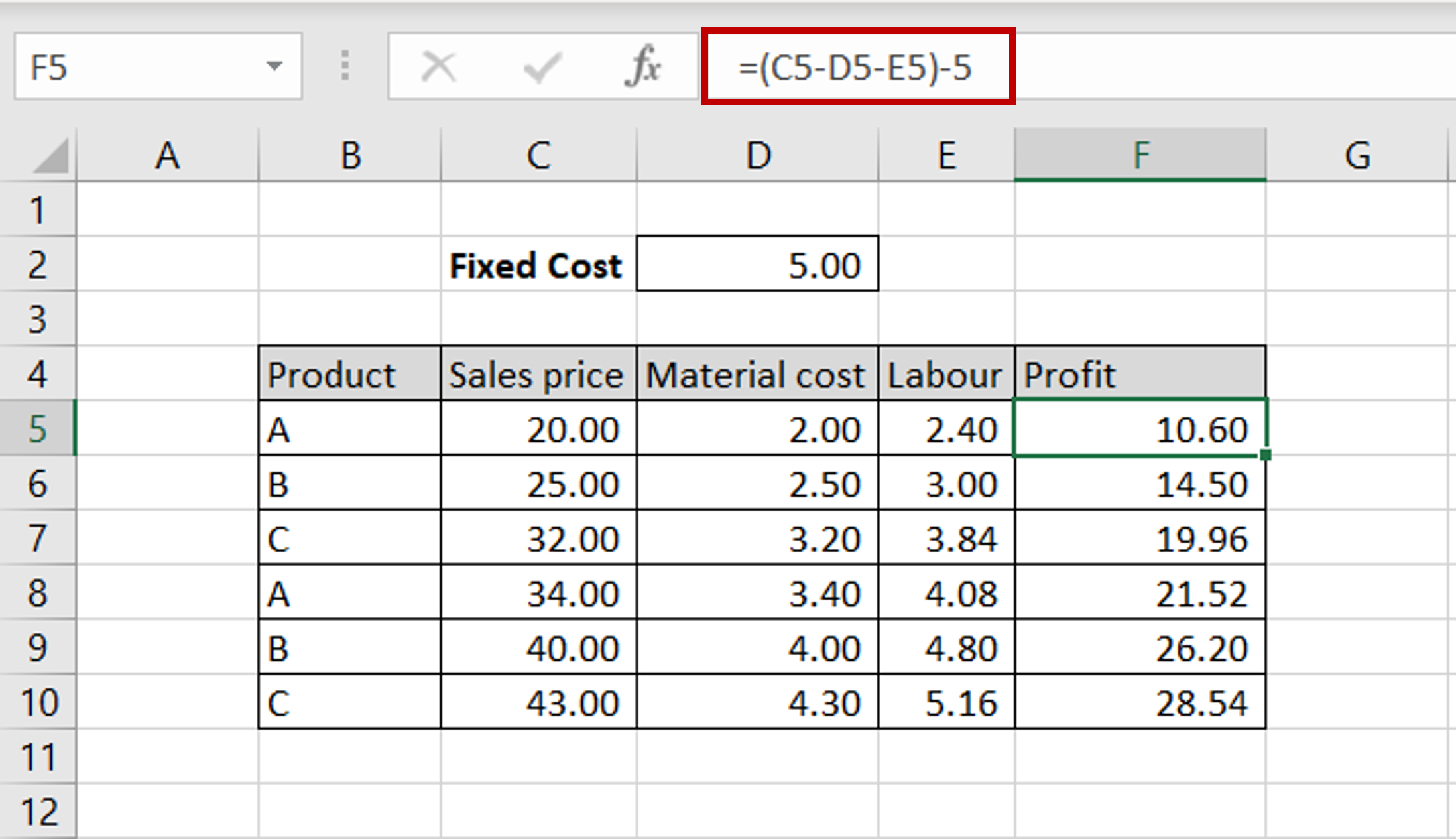Excel is a great tool for performing calculations across many columns and creating complex formulas.  When creating a calculated column, you may need to subtract values from multiple rows and columns.

### Step 1 – Create the formula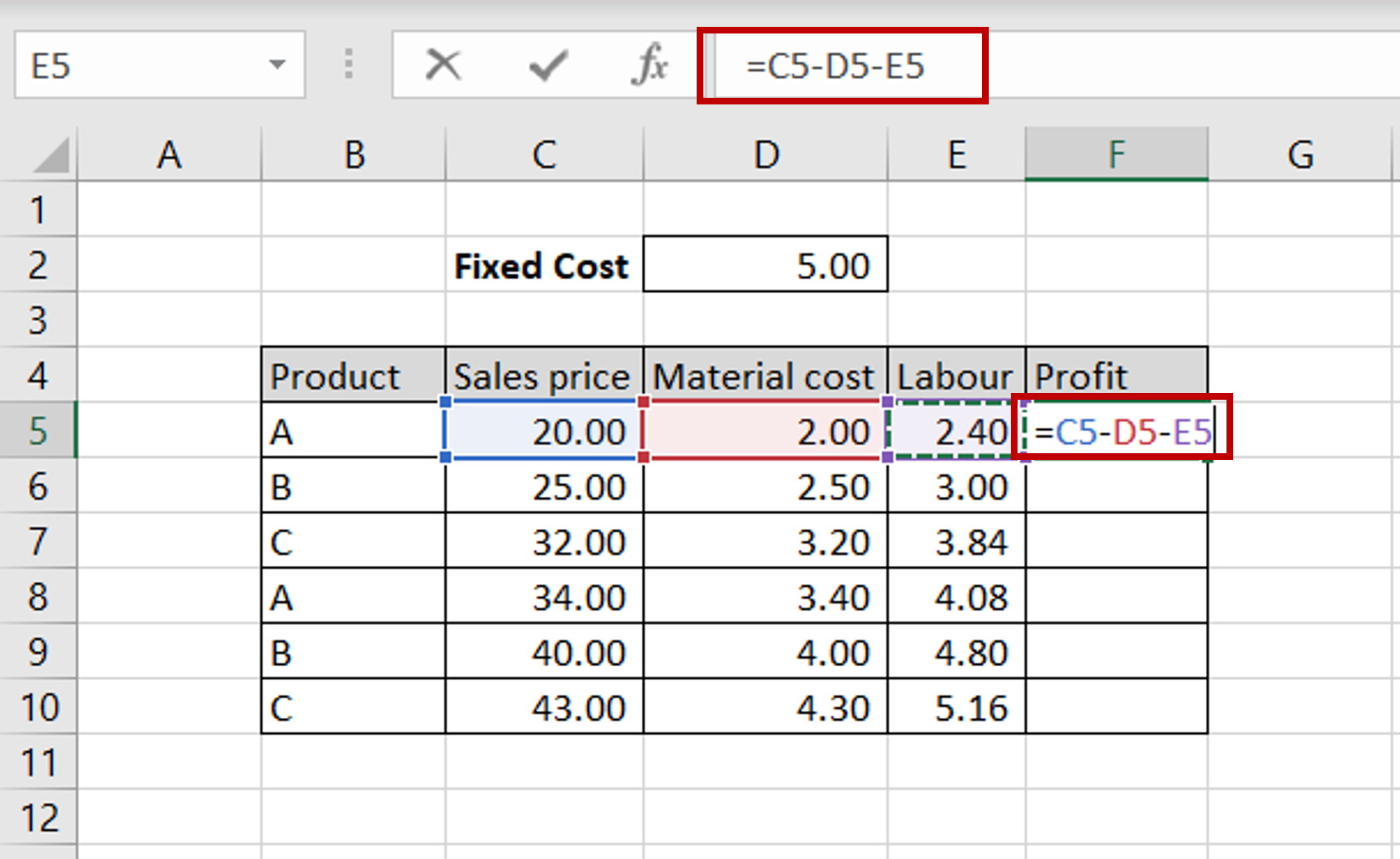– The objective is to find the profit by subtracting Material Cost, Labour and Fixed Cost from the Sales price
– Select the first cell in the ‘Profit’ column
– Type in the formula to subtract the Material Cost and Labour

### Step 2 – Copy the formula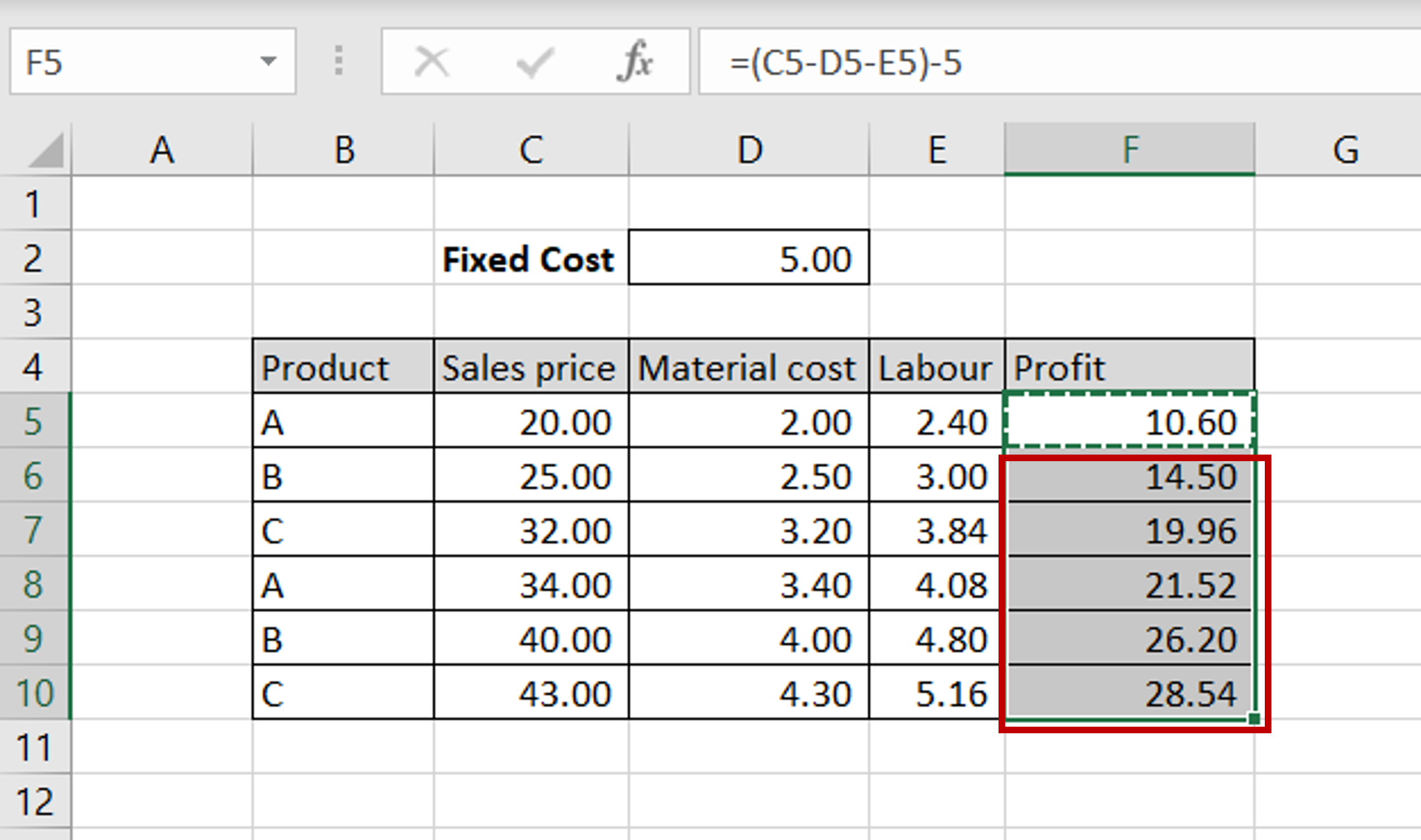– Select the cell with the formula and press Ctrl+C or choose Copy from the context menu (right-click)
– Select the rest of the cells in the column and press Ctrl+V or choose Paste from the context menu (right-click)

### Step 3 – Copy the Fixed Cost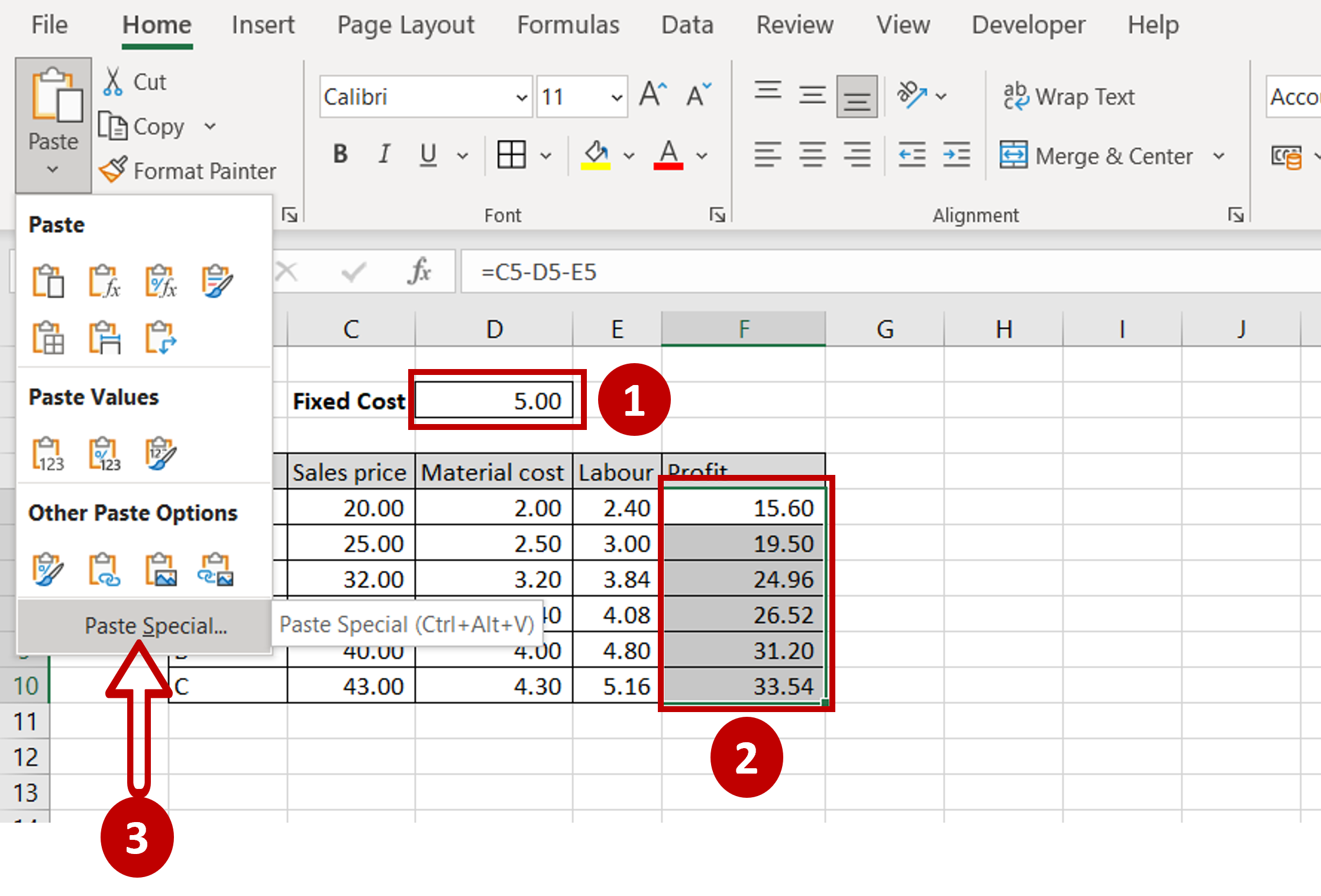– Select the ‘Fixed Cost’ number
– Open the Paste Special window by going to Home > Clipboard > Paste or by right-clicking to open the context menu

### Step 4 – Subtract the Fixed Cost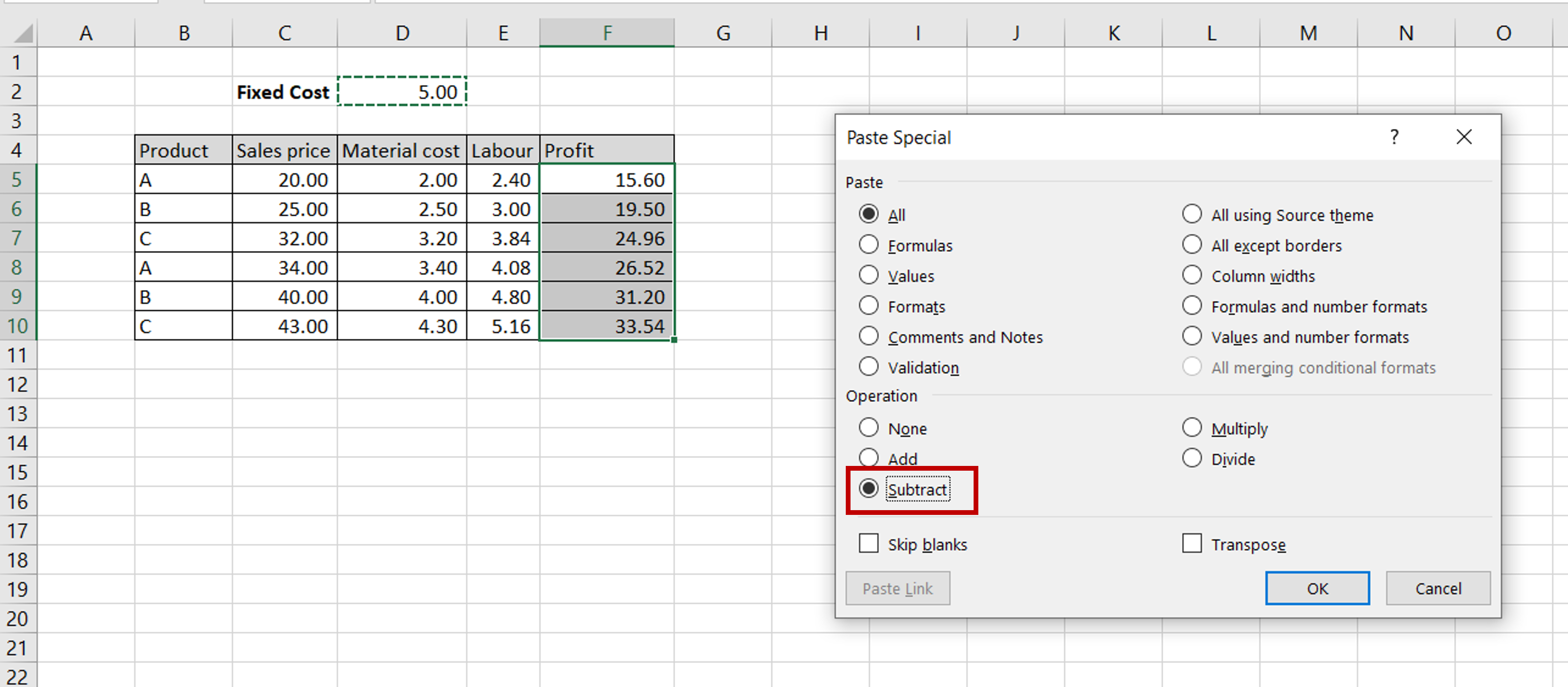– In the Paste Special window, select Subtract
– Click OK

### Step 5 – View the Result– Material Cost, Labour and Fixed Cost have been subtracted from the Sales numbers